# Motion Along A Straight Line

## Introduction

In any scientific experiment that involves moving objects, motion of the objects is defined by various parameters such as speed, velocity and acceleration. It is imperative to learn the meaning of these terms and the relationship between these terms.

A. Speed

One of the most fundamental constants in science is the speed of light in a vacuum. Speed is used in many engineering fields. Let’s consider a car moving on a motorway. The car will cover a certain distance in a certain time. The average speed of the car is defined as the distance moved divided by the time taken. The unit of speed is metres per second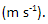Speed does not have an associated direction. It is a scalar quantity.

B. Displacement

Displacement of an object is its change of position. Consider a car travelling 2 km north along a straight road, and then turning round and coming back 1 km. The total distance travelled is 3 km, but the displacement is only 1 km. The displacement is the distance travelled in a straight line in a specified direction from the starting point to the finishing point.

C. Velocity

In ordinary language, there is no difference between the terms ‘speed’ and ‘velocity’. But in science there is an important difference between the two. Velocity is used to define how fast an object is moving and the direction in which it is moving. Velocity is a vector quantity. Speed does not have an associated direction. It is a scalar quantity. The average velocity of the car is defined as the displacement divided by the time taken. The unit of velocity is metres per secondD. Acceleration

The term acceleration is used to describe the changes in velocity of an object. Acceleration is defined as a measure of the rate at which the velocity of a particle is changing. The acceleration of a particle is defined as the change in velocity divided by the time taken. The unit of velocity is metres per second.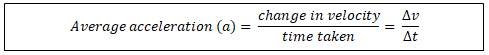E. Equations of Motion

Equations of motion are a series of equations that can be used to give a complete description of the motion of a particle in a straight line. Consider a particle moving along a straight line with a constant acceleration (a). Suppose that its initial velocity, at time t = 0, is u. after a further time (t) its velocity has increased to v. From the definitions of speed, velocity and acceleration, the following equations can be formed. These equations are also called kinematic equations.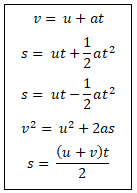In the above equations,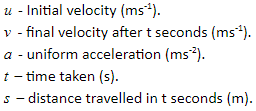F. Displacement-time Graph

In science experiments, it is useful to represent the motion of a particle graphically instead of a series of equations. Consider a car moving in a straight line with a constant velocity so that the car covers equal distance in equal intervals. The following graph illustrates displacement against time. The slope of the line represents the velocity of the car.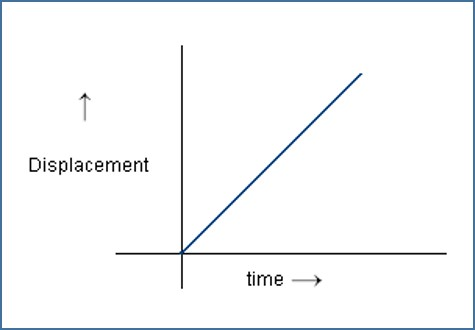Figure 1: Displacement-time Graph

G. Velocity-time Graph

Consider three cases in which a car moving in a straight line with A (constant velocity), B (uniformly accelerated) and C (uniformly retarded) motion. The following graphs illustrate velocity against time for the three cases. The area under the line represents distance travelled by the car.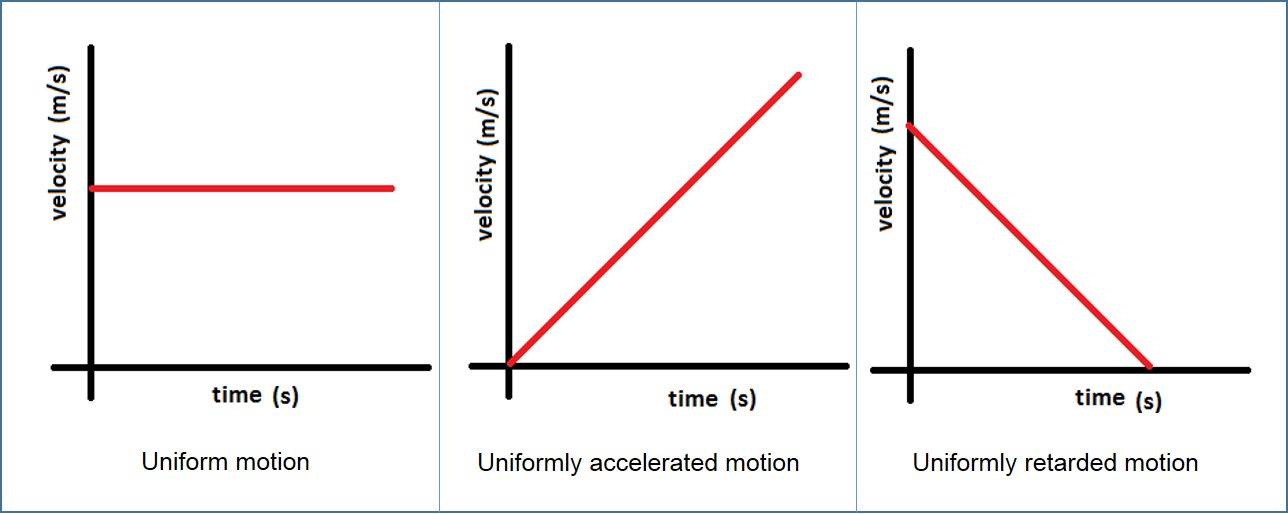Figure 2: Velocity-time Graph

H. Terminal velocity

Terminal velocity is defined as the steady speed achieved by an object freely falling through a gas or liquid. Therefore, terminal velocity is achieved when the speed of a falling object is no longer increasing or decreasing; the object’s acceleration is zero. As an object is falling, force due to gravity acts downward and drag force due to air resistance acts upwards. When the falling speed of the object increases, air resistance also increases. When these forces become equal, then the speed of the falling object will not change, as illustrated in the following diagram.Figure 3: Terminal Velocity

Summary

• The average speed of the car is defined as the distance moved divided by the time taken.
• The displacement is the distance travelled in a straight line in a specified direction from the starting point to the finishing point.
• The average velocity of the car is defined as the displacement divided by the time taken.
• Acceleration is defined as a measure of the rate at which the velocity of a particle is changing.
• Equations of motion are a series of equations that can be used to give a complete description of the motion of a particle in a straight line.
• In a displacement-time graph, the slope of the line represents velocity of a moving object.
• In a velocity-time graph, the area under the line represents the distance covered by a moving object.
• Terminal velocity is defined as the steady speed achieved by an object freely falling through a gas or liquid.